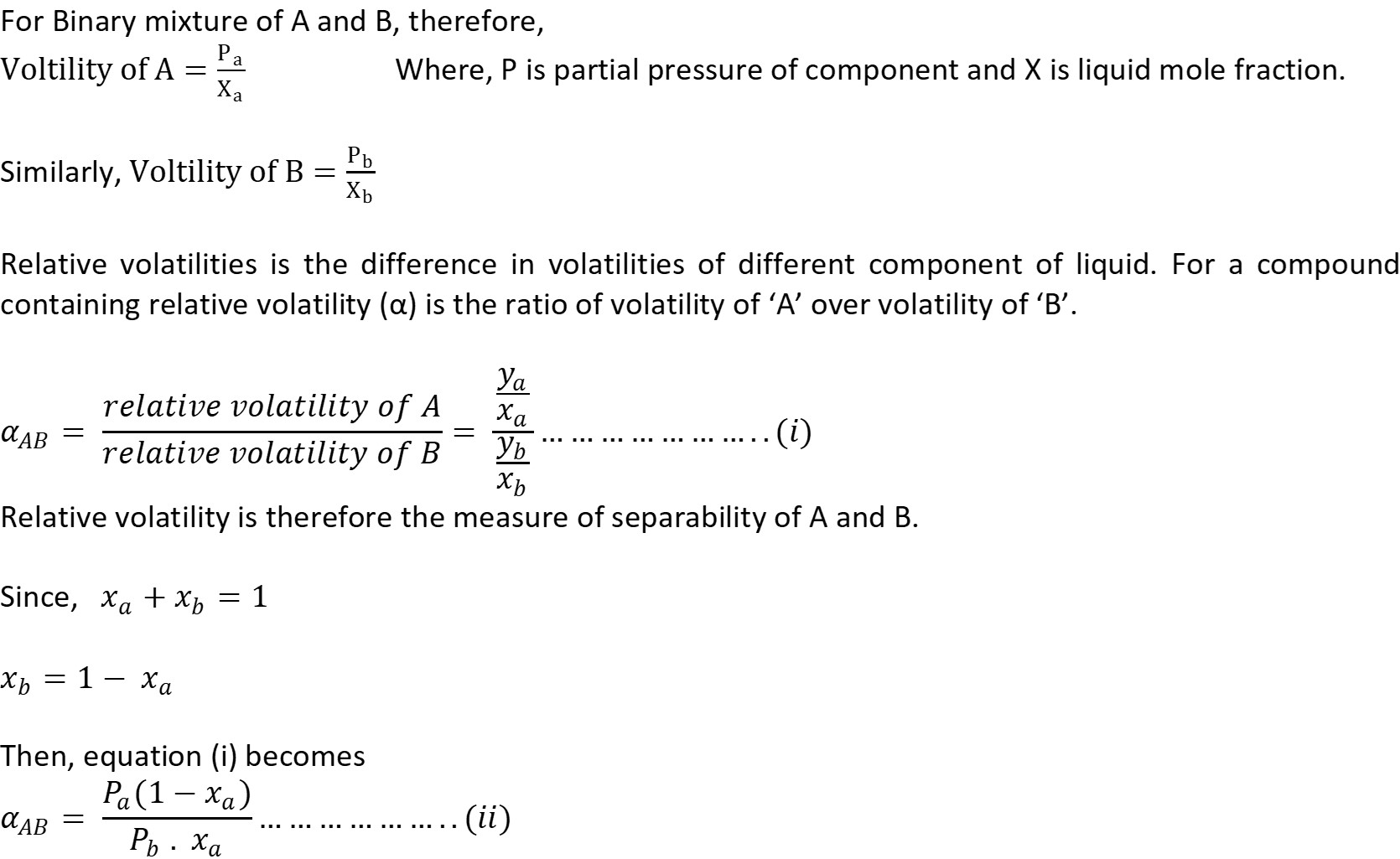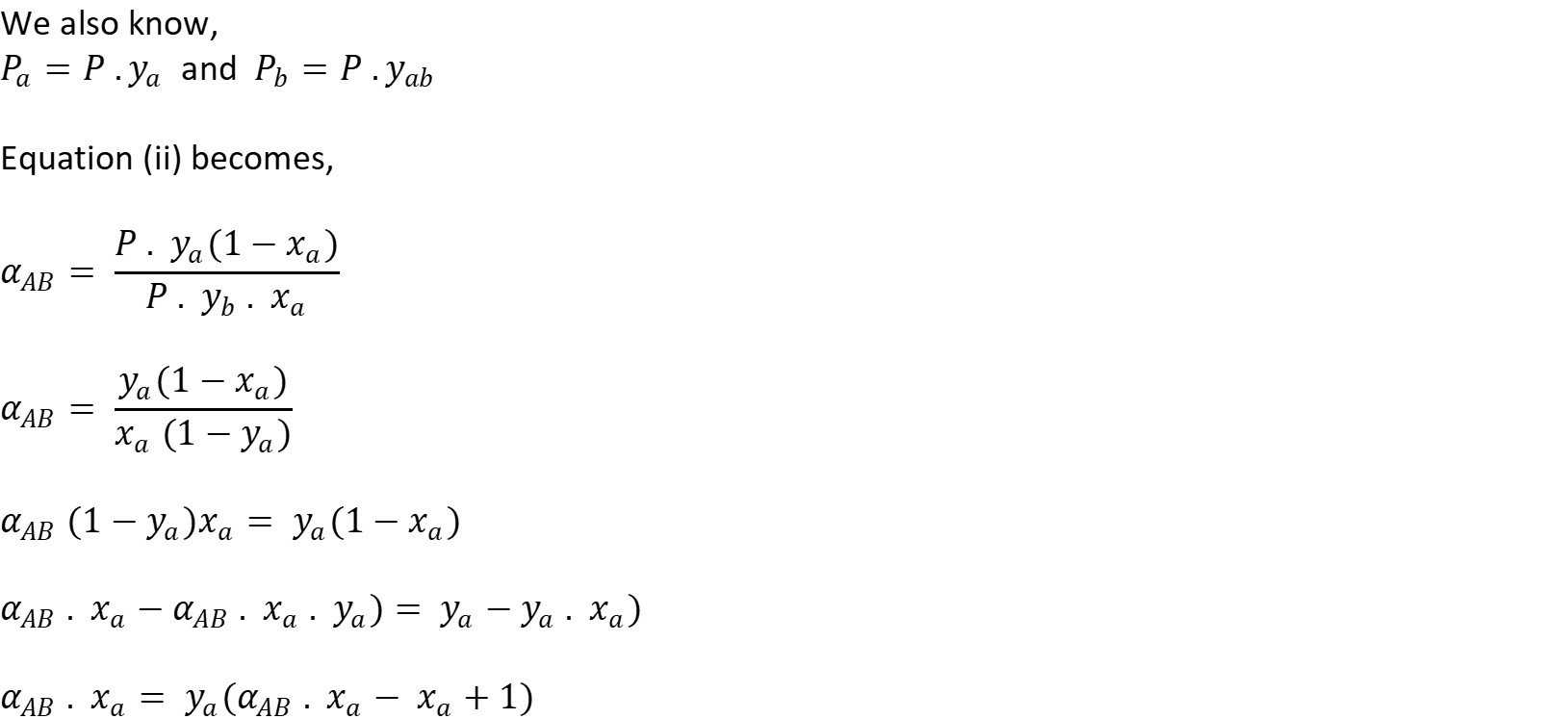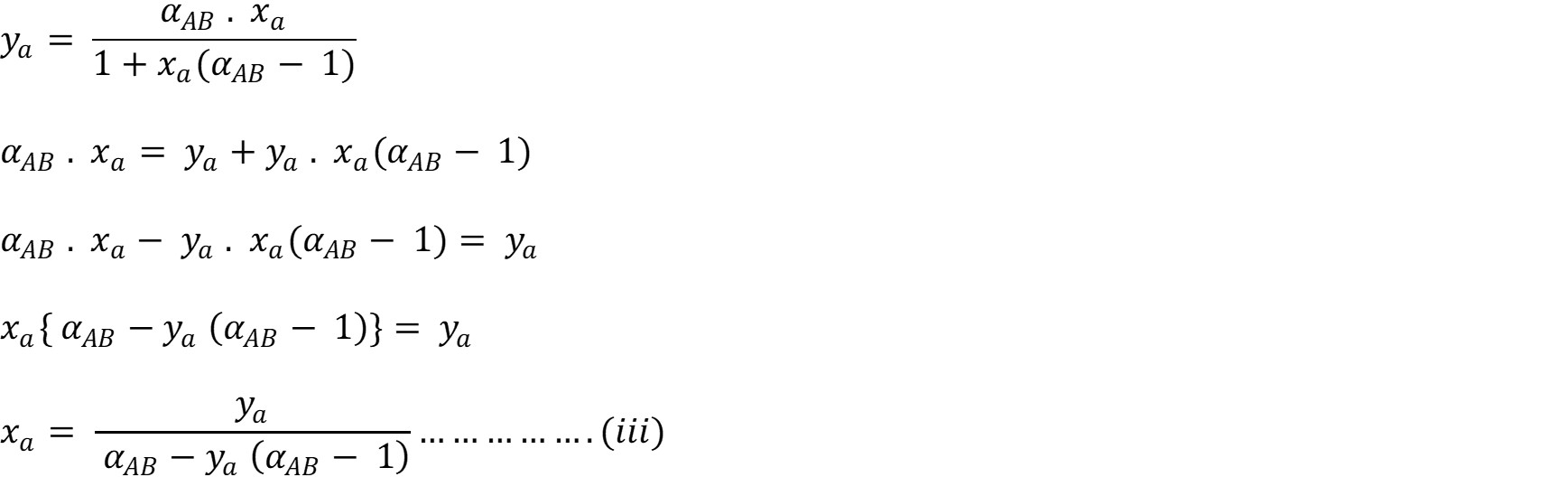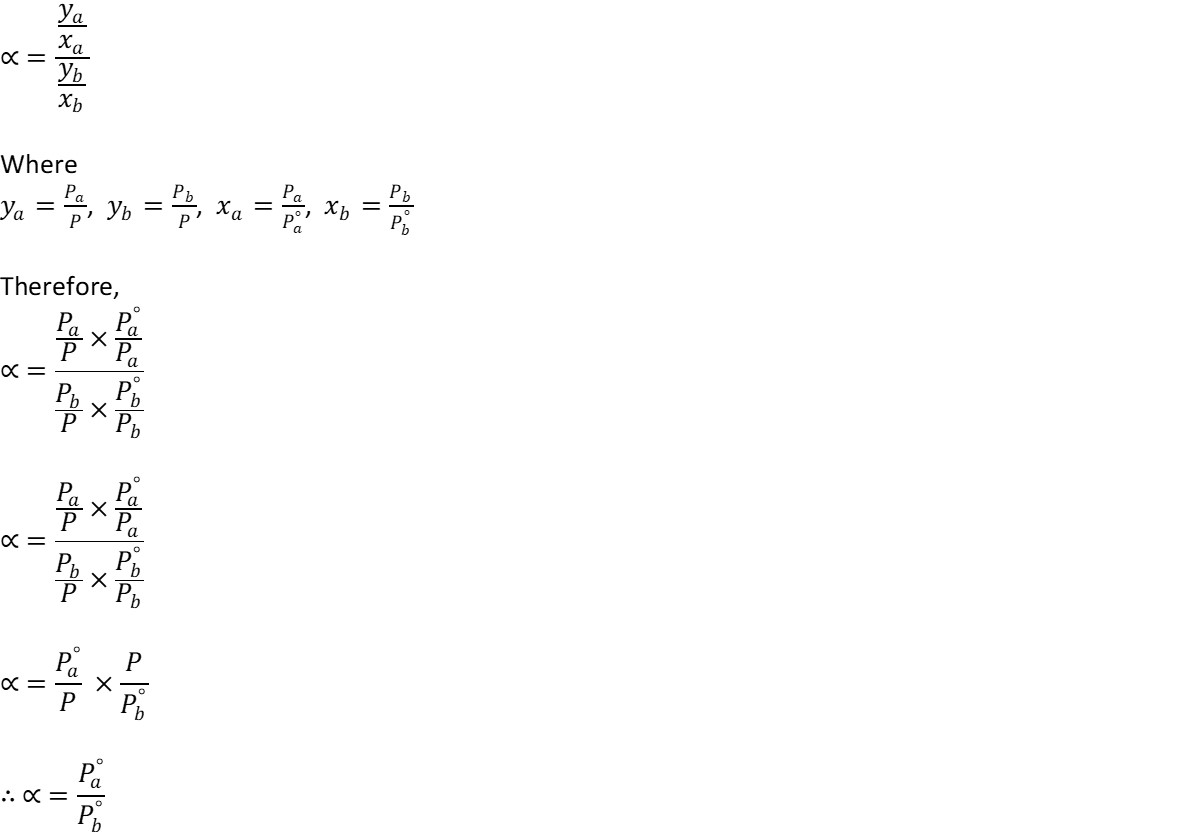# Relative Volatility

In order to separate binary mixtures using distillation process, there must be differences in volatilities of the components. The greater the difference, the easier it is to do so. A measure for this is termed relative volatility. Volatility is a way of expressing relationship between components of vapor Ya and that of liquid Xa in equilibrium. It is defined as ratio of partial pressure of component to mole fraction of component in liquid.This relation enables the components of vapor to be calculated for any defined value of x, if α is known. As α increases above unity, separation becomes much easier. The relation  is useful in calculation of plate enrichment and finds the wide application in multi-component distillation. From the definition of volatility of component, it is seen that for ideal system, the volatility is numerically equal to the vapor pressure of the pure component. Thus, the relative volatility (α) may be expressed as,